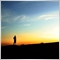# MT4 Coding Problem36

Hello, can you tell me why this coding works:

```extern   double high1=1.256;
double  low1=1.255;
double high2=low1;
double  low2=1.254;
double high3=low2;
double  low3=1.253;
double high4=low3;
double  low4=1.252;
double high5=low4;
double  low5=1.251;
double high6=low5;
double  low6=1.250;
double high7=low6;
double  low7=1.249;
double high8=low7;
double  low8=1.248;
double high9=low8;
double  low9=1.247;```

And why this coding does not work ?

```extern double distance=0.001;

extern   double high1=1.256;
double  low1=high1-distance;
double high2=low1;
double  low2=high2-distance;
double high3=low2;
double  low3=high3-distance;
double high4=low3;
double  low4=high4-distance;
double high5=low4;
double  low5=high5-distance;
double high6=low5;
double  low6=high6-distance;
double high7=low6;
double  low7=high7-distance;
double high8=low7;
double  low8=high8-distance;
double high9=low8;
double  low9=high9-distance;```

Thank you20233

1. Why did you post your MT4 question in the Root / MT5 EA section instead of the MQL4 section, (bottom of the Root page?)
General rules and best pratices of the Forum. - General - MQL5 programming forum
Next time post in the correct place. The moderators will likely move this thread there soon.

2. ```extern double distance=0.001;

extern   double high1=1.256;
double  low1=high1-distance;```
Global and static variables work exactly the same way in MT4/MT5/C/C++.
1. They are initialized once on program load.
2. They don't update unless you assign to them.
3. In C/C++ you can only initialize them with constants, and they default to zero. In MTx you should only initialize them with constants. There is no default in MT5 (or MT4 with strict which you should always use.)

MT4/MT5 actually compiles with non-constants, but the order that they are initialized is unspecified and don't try to use any price or server related functions in OnInit (or on load,) as there may be no connection/chart yet:

1. Terminal starts.
2. Indicators/EAs are loaded. Static and globally declared variables are initialized. (Do not depend on a specific order.)
3. OnInit is called.
4. For indicators OnCalculate is called with any existing history.
5. Human may have to enter password, connection to server begins.
6. New history is received, OnCalculate called again.
7. New tick is received, OnCalculate/OnTick is called. Now TickValue, TimeCurrent, account information and prices are valid.
4. Unlike indicators, EAs are not reloaded on chart change so you must reinitialize them, if necessary. Think symbol/TF change.
external static variable - Inflation - MQL4 programming forum

Initialize your calculations in OnInit after the input variables are set.36

Thank You William